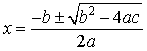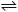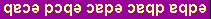CH223 - 11:00                            EXAM-2A                               Practice

 Information: gas constant:  R = 0.08206 L·atm/mole·K = 8.314 J/mole·K Avogadro's number:  NA = 6.022 ´ 1023/mol Q-dependence of free energy change:  DG  =  DGo  + RT ln(Q) normal freezing point of water:   0°C = 273K water ionization constant (25°C):  Kw = 1.00 ´ 10-14 pressure:  1 atm = 760 mmHg = 760 torr log conversion:  ln(x) = 2.303 log(x) quadratic equation:   ax2 + bx + c = 0,Multiple choice:  select one answer for each question:

1.  The pH of a buffer solution that consists of 0.58 M boric acid (H3BO3 , pKa = 9.24) and 0.27 M sodium borate (NaH2BO3) is:

 a) 8.70 b) 9.34 c) 8.46 d) 9.57 e) 8.91

2.  The best criterion for the spontaneity of a chemical reaction at constant T and P is the sign of

 a) DH b) DH° c) TDS d) DG e) DG°

3.   Find DG° for the reaction at 298K:    I2(g) + Cl2(g)2 ICl(g),     DH° = -26.9 kJ,    DS° =  11.3 J/K

 a) 102 kJ b) -30.3 kJ c) 50.6 kJ d) +18.4 kJ e) -50.6 kJ

4.  The solubility of PbSO4 (Ksp = 1 ´ 10-8 ) in water is
 a) 1 ´ 10-4 M b) 1 ´ 10-7 M c) 1 ´ 10-5 M d) 1 ´ 10-8 M e) 1 ´ 10-6 M

5.  In which of the following reactions will DS° be positive?

 1.   N2O4(g)  ®  2NO2(g) 2.   CaO(s) + CO2(g) ®  CaCO3(s) 3.   sublimation of CO2 a) 1 only b) 2 only c) 1 and 2 only d) 1 and 3 only e) 1, 2 and 3

6. The equation associated with Kf for the complex ion Ag(NH3)2+ is

 a) Ag(NH3)2+(aq)Ag+(aq) + 2NH3(aq) b) Ag+(aq) + 2NH3(aq)Ag(NH3)2+(aq) c) Ag(NH3)2+(aq)Ag+(g) + 2NH3(g) d) Ag+(g) + 2NH3(g)Ag(NH3)2+(aq) e) Ag+(aq) + N2(g) + 3H2(g)Ag(NH3)2+(aq)

7.   The pH after mixing 0.00020 mole of HCl and 0.00018 mole of NaOH in 1.000 L of solution is:

 a) 4.70 b) 5.00 c) 5.30 d) 5.60 e) 9.00

8.  A chemical equilibrium that favors reactants at high temperature and products at low temperature has

 a) DHo > 0,  DSo > 0 b) DHo > 0,  DSo < 0 c) DHo < 0,  DSo < 0 d) DHo < 0,  DSo > 0 e) none of the above

9.  Calculate the equilibrium constant Kp for the reaction at 500K:

 Cu(s) + H2O(g)CuO(s) + H2(g),      DG°500K = 110.6 kJ a) 2.4 ´ 10-13 b) 5.6 ´ 10-12 c) 0.97 d) 3.5 ´ 10-11 e) 2.8 ´ 10-12

10.  Calculate the solubility of Cu(OH)2 (Ksp =1 ´10-20) in a solution buffered at pH = 6.00.

 a) 1 ´10-6 M b) 1 ´10-14 M c) 1 ´10-8 M d) 1 ´10-4 M e) 1 ´10-10 M

 Questions 11-15:  Titrate 64 mL of 0.200M weak acid HA (Ka = 1 ´ 10-8 ) with 0.200M NaOH.

11.  The equilibrium concentrations at the equivalence point satisfy

 a) [HA] < [A- ] b) [HA] = [A- ] c) [HA] > [A- ]

12.  The volume of NaOH needed to reach the buffer point is

 a) 64 mL b) 16 mL c) 32 mL d) 48 mL e) 72 mL

13.   The pH at the buffer point is

 a) 4 b) 7 c) 9 d) 6 e) 8

14.  At pH = 7 the quotient [A- ] / [HA] is

 a) 0.01 b) 0.10 c) 1 d) 10 e) 100

15.  The pH at the equivalence point satisfies

 a) pH < 7 b) pH = 7 c) pH > 7

 Questions 16-20:  Titrate 60 mL of 0.50 M acid H2A (Ka1 = 1 ´ 10-3 , Ka2 = 1 ´ 10-9 ) with 0.50 M NaOH .

16. The volume of NaOH needed to reach the first equivalence point is:

 a) 90 mL b) 30 mL c) 75 mL d) 60 mL e) 45 mL

17.  The pH at the first equivalence point is:

 a) 7 b) 9 c) 3 d) 8 e) 6

18.    Equilibrium concentrations at the first equivalence point satisfy:

 a) [HA- ] > [A2- ] > [H2A] b) [H2A] = [HA- ] > [A2- ] c) [HA- ] > [A2- ] = [H2A] d) [A2- ] = [HA- ] > [H2A] e) [HA- ] > [H2A] > [A2- ]

19.  Equilibrium concentrations at pH = 4 satisfy:

 a) [HA- ] > [H2A] > [A2- ] b) [A2- ] = [HA- ] > [H2A] c) [HA- ] > [A2- ] = [H2A] d) [H2A] = [HA- ] > [A2- ] e) [HA- ] > [A2- ] > [H2A]

20.  Equilibrium concentrations at the second buffer point satisfy:

 a) [A2- ] > [HA- ] > [H2A] b) [A2- ] = [HA- ] > [H2A] c) [HA- ] > [H2A] > [A2- ] d) [HA- ] > [A2- ] = [H2A] e) [H2A] = [HA- ] > [A2- ]

 KEY: